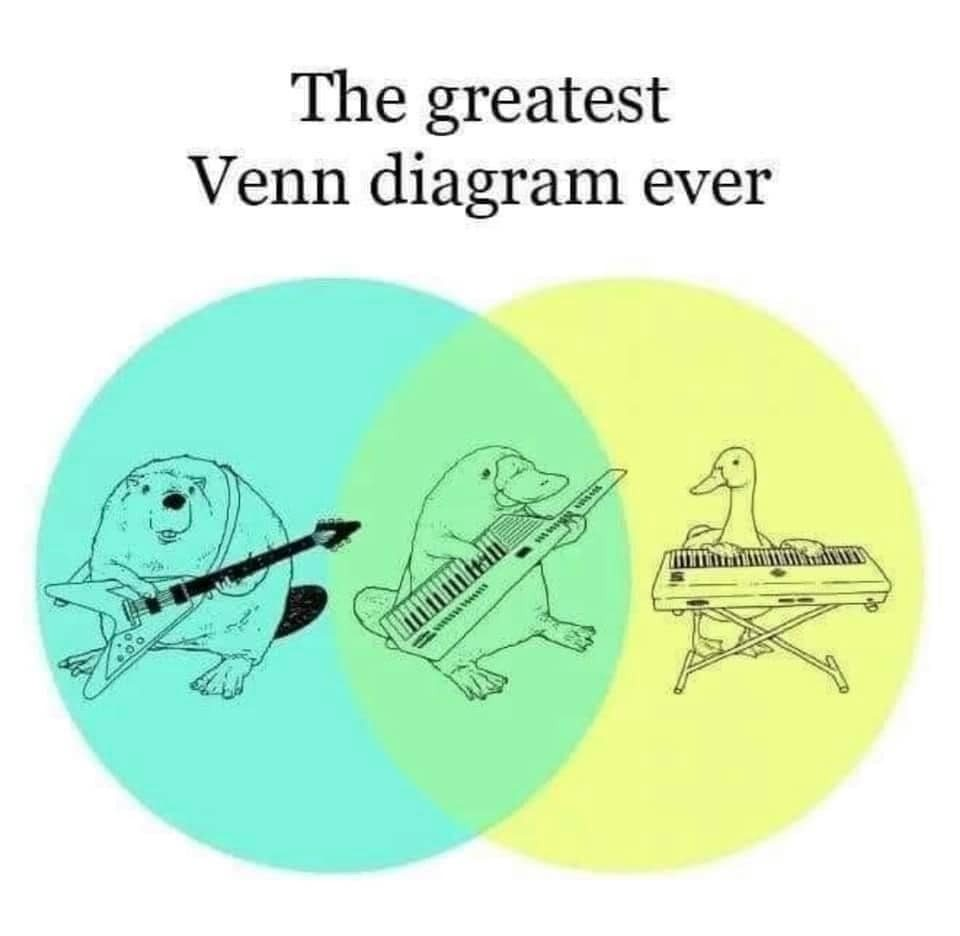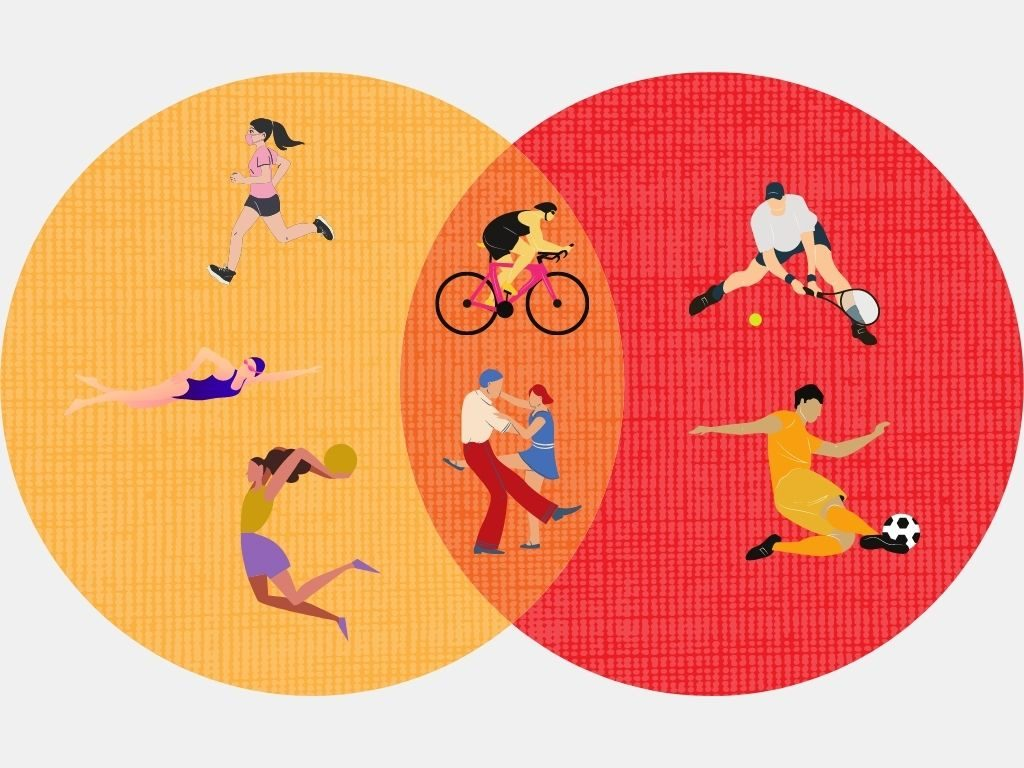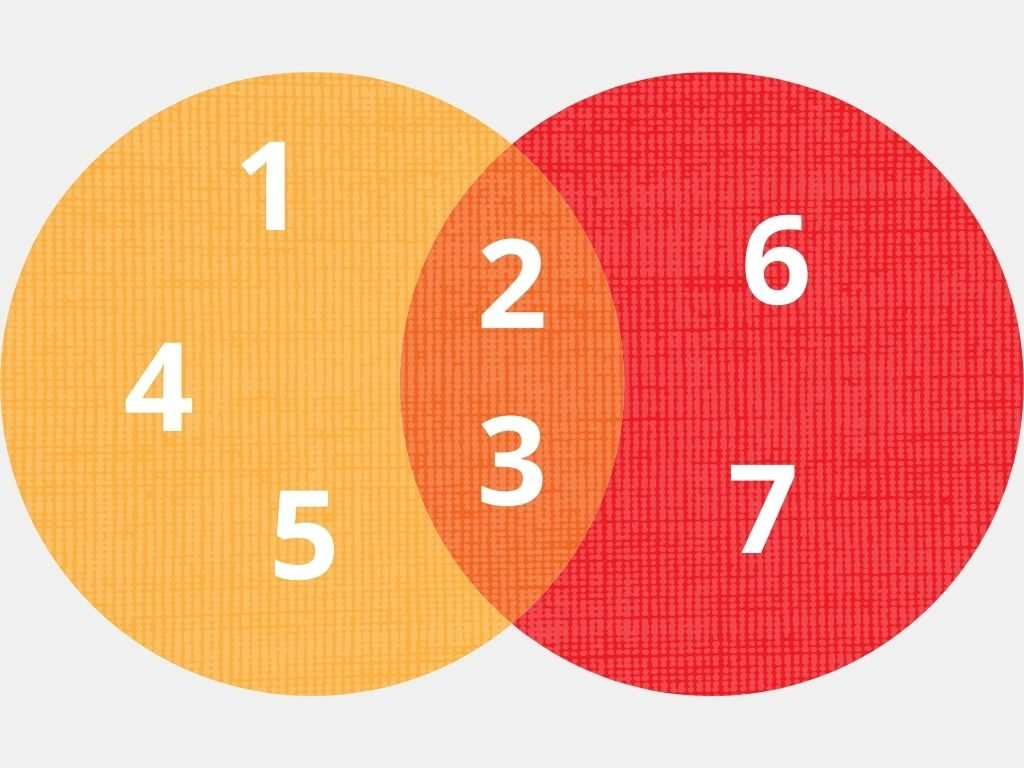# Union and Intersection Calculator

Created by Maciej Kowalski, PhD candidate
Reviewed by Anna Szczepanek, PhD and Steven Wooding
Last updated: Jun 08, 2022

Welcome to Omni's union and intersection calculator, where we'll learn how to find A∪B and A∩B, i.e., A union B and A intersection B. Obviously, apart from learning the symbols for set operations, we'll formally define the union and intersection of sets to see what the difference is. In short, it all boils to whether we want the elements to be in any of the sets or all of them. Still, the comparison of union vs. intersection deserves its very own section, which we further support with some union and intersection examples.

## Union and intersection of sets and their symbols

Before we move to operations, let's see the formal set definition.

💡 A set is a collection of distinct elements.

Observe how general the concept is. In particular:

We often want to compare two or more sets: see which elements belong to one and not the other, or which are common to both. This takes us to set operations. There are quite a few of those, but you've visited us to see the union and intersection calculator, so we'll focus on those two.

💡 The union of two sets is the set containing all elements that belong to at least one of the initial sets. The math union symbol is ∪, i.e., we read A∪B as A union B or the union of A and B.

💡 The intersection of two sets is the set containing all elements that belong to both the first and the second set. The math intersection sign is ∩, i.e., we read A∩B as A intersection B or the intersection of A and B.

Alright, we've defined the union and intersection formally, but let's maybe spare a few sentences to translate them to everyday language. However, it so happens that it's helpful to study the concepts by comparing the similarities and differences between the union and intersection of sets.

## Union vs. intersection

Before we see some union and intersection examples, let's try to understand the two operations by contrasting them. For that, below, we've defined the union and intersection again, but using everyday language.

The union of sets A and B gathers all elements that fall in A, in B, or in both. As such, it's always larger than or equal to either of the two (i.e., it's a superset of both).

On the other hand, the intersection of sets A and B gathers elements common to both, i.e., all that fall both in A and in B. As such, it's always smaller than or equal to either of the two (i.e., it's a subset of both).

In particular, the intersection always lives inside the union. What is more, they are equal if and only if the two underlying sets are equal. And to explain the differences some more, let's see what happens if one of the sets is wholly contained in the other. Then, according to the explanation above:

• A union B is the larger of the two; and
• A intersection B is the smaller of the two.

Furthermore, observe that it doesn't really matter in what order we take the sets: A∪B is the same as B∪A, and A∩B is the same as B∩A. In math, we call this property the commutativity of the union and intersection of sets.

As a matter of fact, the two operations have more properties in common. For instance, even though we've defined the union and intersection of only two sets, we can easily extend it to more. To be precise, A₁∪A₂∪A₃∪...∪Aₙ and A₁∩A₂∩A₃∩...∩Aₙ simply denote the collections of elements belonging to, respectively, any and all of the listed sets. In fact, this notation makes sense because both operations satisfy the associative property:

• A∪(B∪C) = (A∪B)∪C

• A∩(B∩C) = (A∩B)∩C

At this point, you may feel that the union and intersection of sets are quite similar to the two basic arithmetic operations: addition and multiplication. Indeed, scientists often refer to the union symbol as sum and to the intersection sign as product.

The similarities may help you wonder if we have here an equivalent of another arithmetic law: the distributive property of multiplication over addition. And indeed we do! What is more, while with numbers, it only works one way (i.e., multiplication over addition), in set theory, it works both ways: union over intersection and intersection over union.

• A∪(B∩C) = (A∪B)∩(A∪C)

• A∩(B∪C) = (A∩B)∪(A∩C)

Note how we used brackets to make sure we don't mix the two operations.

Lastly, let us mention that the comparison of union vs. intersection is best portrayed by so-called Venn diagrams. They are graphic representations using circles or ellipses for each of the sets, with the shapes intersecting to show which elements are common and which are not.Don't worry; we'll see a proper number-based diagram very soon, along with an implementation of all the above theory to practice: it's time for union and intersection examples!

## Example: using the union and intersection calculator

Suppose that Amy and Mark want to lose some weight after the pandemic lockdowns and restrictions. Besides taking special care of their diet, they decide to take up a physical activity; it's just a matter of choosing the right one.

Amy likes jogging, cycling, dancing, swimming, and basketball. On the other hand, Mark enjoys dancing, tennis, soccer, and cycling. Let's use Omni's union and intersection calculator to find what the couple may like.

To begin with, our tool (like any other calculator) prefers numbers. Therefore, let us translate all the activities listed above to consecutive integers:

• 1 for jogging;
• 2 for cycling;
• 3 for dancing;
• 4 for swimming;
• 5 for basketball;
• 6 for tennis; and
• 7 for soccer.

Looking at the union and intersection calculator, we see that first, we need to tell the tool what we're dealing with. For that, we select 2 under "Number of sets" (since we have two people) and "individual entries" under "Input form" (since we'll list the activities one by one).

That'll trigger two sections underneath: one for set A and one for set B. There, respectively, we input the numbers corresponding to the activities that Amy and Mark enjoy, so in the first, we input:

• 1, 2, 3, 4, 5,

and in the second, we write:

• 3, 6, 7, 2.

(Note how, initially, the union and intersection calculator shows only three variable fields in each section. However, new ones appear once you begin inputting the values. In total, you can have sets with up to ten elements each.)

Once you give the last entry, the union and intersection calculator will spit out the answer underneath. There, we'll see:

• The elements exclusive to A (i.e., those that Amy likes, but Mark doesn't);
• The elements exclusive to B (i.e., those that Mark likes, but Amy doesn't);
• A union B (i.e., activities that at least one of them likes); and
• A intersection B (i.e., activities that both of them like).

However, before we reveal the answer, let's find it ourselves. Recall Venn diagrams from : we'll draw one to illustrate the union and intersection of the sets in question.

If we put Amy's interests in a yellow circle and Marks in a red one, we'll get something like this:From the picture, it's now easy to read the union and intersection of the sets:

• Amy likes jogging, swimming, and basketball, but Mark doesn't;
• Mark likes tennis and soccer, but Amy doesn't;
• At least one of them likes jogging, swimming, basketball, cycling, dancing, tennis, and soccer; and
• Both of them like cycling and dancing.

And just in case you'd like to see the diagram with the integers corresponding to the activities, there it is:Make sure to experiment with Omni's union and intersection calculator to discover all its functionalities. In particular, check out the variant using interval notation, i.e., the infinite subsets of the number line.

## FAQ

### What is the difference between union and intersection?

On the one hand, the union gathers all the elements occurring in at least one of the sets. As such, all of them are its subsets. For example, the union of two sets with one entirely contained in the other is equal to the larger one.

On the other hand, the intersection gathers all the elements common to each and every one of the sets. As such, it's a subset of each of them. For instance, the intersection of two sets with one entirely contained in the other is equal to the smaller one.

### How do I find the union and intersection of intervals?

To find the union and intersection of two intervals, you need to:

1. Draw the number line.
2. Mark the endpoints of the first interval.
3. Color the line between those points.
4. Mark the endpoints of the second interval.
5. Color the line between those points using a different color.
6. The left endpoint of:
• The union is the leftmost of the left interval endpoints; and
• The intersection is the rightmost of the left interval endpoints.
7. The right endpoint of:
• The union is the rightmost of the right interval endpoints; and
• The intersection is the leftmost of the right interval endpoints.
8. For the intersection, if the left endpoint is larger, the intersection is empty.
9. Mark the endpoints as open or closed, depending on the initial data.

### How do I find the union and intersection of two sets?

To find the union and intersection of two sets, you need to:

1. List all elements from the first set.
2. Add the elements from the second set.
3. Cross out any repetitions (i.e., leave one copy of each).
4. For every element, check if it's in the sets:
• The union gathers those that appear in at least one; and
• The intersection gathers those that appear in both.
5. Enjoy having found the union and intersection of two sets.

### Are the union and intersection operations distributive?

Yes. In other words, for any sets A, B, and C, we have:

• A∪(B∩C) = (A∪B)∩(A∪C)
• A∩(B∪C) = (A∩B)∪(A∩C)

### Are the union and intersection operations commutative?

Yes. In other words, for any two sets A and B, we have:

• A∪B = B∪A
• A∩B = B∩A
Maciej Kowalski, PhD candidate
Number of sets
2
Input form
Individual entries
Set A
Entry #1
Entry #2
Entry #3
Set B
Entry #1
Entry #2
Entry #3
People also viewed…

### BMR - Harris-Benedict equation

Harris-Benedict calculator uses one of the three most popular BMR formulas. Knowing your BMR (basal metabolic weight) may help you make important decisions about your diet and lifestyle.

### GCF and LCM

GCF and LCM determines the greatest common factor and least common multiple of 2-6 numbers.

### Heron's formula

What's the Heron's area formula? What's the Heron's formula proof? Find out with this Heron's formula calculator!

### Sunbathing

Do you always remember to put on sunscreen before going outside? Are you sure that you use enough? The Sunbathing Calculator ☀ will tell you when's the time to go back under an umbrella not to suffer from a sunburn!